## Thermal Expansion of Solids and Liquids

### Learning Objectives

By the end of this section, you will be able to:

• Define and describe thermal expansion.
• Calculate the linear expansion of an object given its initial length, change in temperature, and coefficient of linear expansion.
• Calculate the volume expansion of an object given its initial volume, change in temperature, and coefficient of volume expansion.
• Calculate thermal stress on an object given its original volume, temperature change, volume change, and bulk modulus.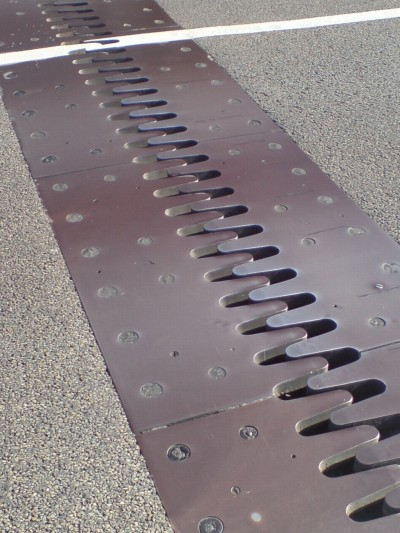Figure 1. Thermal expansion joints like these in the Auckland Harbour Bridge in New Zealand allow bridges to change length without buckling. (credit: Ingolfson, Wikimedia Commons)

The expansion of alcohol in a thermometer is one of many commonly encountered examples of thermal expansion, the change in size or volume of a given mass with temperature. Hot air rises because its volume increases, which causes the hot air’s density to be smaller than the density of surrounding air, causing a buoyant (upward) force on the hot air. The same happens in all liquids and gases, driving natural heat transfer upwards in homes, oceans, and weather systems. Solids also undergo thermal expansion. Railroad tracks and bridges, for example, have expansion joints to allow them to freely expand and contract with temperature changes.

What are the basic properties of thermal expansion? First, thermal expansion is clearly related to temperature change. The greater the temperature change, the more a bimetallic strip will bend. Second, it depends on the material. In a thermometer, for example, the expansion of alcohol is much greater than the expansion of the glass containing it.

What is the underlying cause of thermal expansion? As is discussed in Kinetic Theory: Atomic and Molecular Explanation of Pressure and Temperature, an increase in temperature implies an increase in the kinetic energy of the individual atoms. In a solid, unlike in a gas, the atoms or molecules are closely packed together, but their kinetic energy (in the form of small, rapid vibrations) pushes neighboring atoms or molecules apart from each other. This neighbor-to-neighbor pushing results in a slightly greater distance, on average, between neighbors, and adds up to a larger size for the whole body. For most substances under ordinary conditions, there is no preferred direction, and an increase in temperature will increase the solid’s size by a certain fraction in each dimension.

### Linear Thermal Expansion—Thermal Expansion in One Dimension

The change in length ΔL is proportional to length L. The dependence of thermal expansion on temperature, substance, and length is summarized in the equation ΔαLΔT,where ΔL is the change in length L, ΔT is the change in temperature, and α is the coefficient of linear expansion, which varies slightly with temperature.

Table 1 lists representative values of the coefficient of linear expansion, which may have units of 1/ºC or 1/K. Because the size of a kelvin and a degree Celsius are the same, both α and ΔT can be expressed in units of kelvins or degrees Celsius. The equation ΔαLΔT is accurate for small changes in temperature and can be used for large changes in temperature if an average value of α is used.

Table 1. Thermal Expansion Coefficients at 20ºC
Material Coefficient of linear expansion α(1/ºC) Coefficient of volume expansion β(1/ºC)
Solids
Aluminum 25 × 10 6 75 × 10 6
Brass 19 × 10 6 56 × 10 6
Copper 17 × 10 6 51 × 10 6
Gold 14 × 10 6 42 × 10 6
Iron or Steel 12 × 10 6 35 × 10 6
Invar (Nickel-iron alloy) 0.9 × 10 6 2.7 × 10 6
Lead 29 × 10 6 87 × 10 6
Silver 18 × 10 6 54 × 10 6
Glass (ordinary) 9 × 10 6 27 × 10 6
Glass (Pyrex®) 3 × 10 6 9 × 10 6
Quartz 0.4 × 10 6 1 × 10 6
Concrete, Brick ~12 × 10 6 ~36 × 10 6
Marble (average) 2.5 × 10 6 7.5 × 10 6
Liquids
Ether 1650 × 10 6
Ethyl alcohol 1100 × 10 6
Petrol 950 × 10 6
Glycerin 500 × 10 6
Mercury 180 × 10 6
Water 210 × 10 6
Gases
Air and most other gases at atmospheric pressure 3400 × 10 6

### Example 1. Calculating Linear Thermal Expansion: The Golden Gate Bridge

The main span of San Francisco’s Golden Gate Bridge is 1275 m long at its coldest. The bridge is exposed to temperatures ranging from 15ºC to 40ºC. What is its change in length between these temperatures? Assume that the bridge is made entirely of steel.

#### Strategy

Use the equation for linear thermal expansion ΔLαLΔT to calculate the change in length , ΔL. Use the coefficient of linear expansion, α, for steel from Table 1, and note that the change in temperature, ΔT, is 55ºC.

#### Solution

Plug all of the known values into the equation to solve for ΔL.

$\Delta{L}=\alpha{L}\Delta{L}=\left(\frac{12\times10^{-6}}{^{\circ}\text{C}}\right)\left(1275\text{ m}\right)\left(55^{\circ}\text{C}\right)=0.84\text{ m}\\$

#### Discussion

Although not large compared with the length of the bridge, this change in length is observable. It is generally spread over many expansion joints so that the expansion at each joint is small.

## Thermal Expansion in Two and Three Dimensions

Objects expand in all dimensions, as illustrated in Figure 2. That is, their areas and volumes, as well as their lengths, increase with temperature. Holes also get larger with temperature. If you cut a hole in a metal plate, the remaining material will expand exactly as it would if the plug was still in place. The plug would get bigger, and so the hole must get bigger too. (Think of the ring of neighboring atoms or molecules on the wall of the hole as pushing each other farther apart as temperature increases. Obviously, the ring of neighbors must get slightly larger, so the hole gets slightly larger).

### Thermal Expansion in Two Dimensions

For small temperature changes, the change in area ΔA is given by Δ= 2αAΔT, where ΔA is the change in area A, ΔT is the change in temperature, and α is the coefficient of linear expansion, which varies slightly with temperature.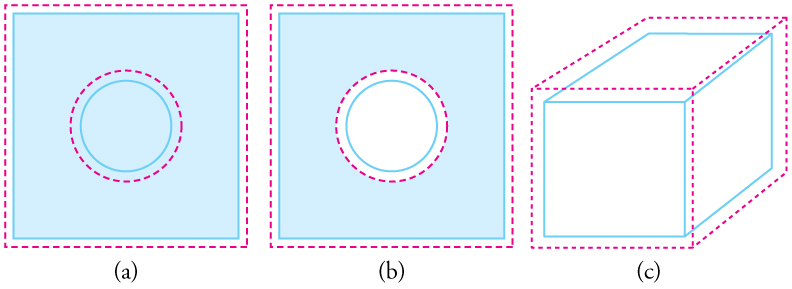Figure 2. In general, objects expand in all directions as temperature increases. In these drawings, the original boundaries of the objects are shown with solid lines, and the expanded boundaries with dashed lines. (a) Area increases because both length and width increase. The area of a circular plug also increases. (b) If the plug is removed, the hole it leaves becomes larger with increasing temperature, just as if the expanding plug were still in place. (c) Volume also increases, because all three dimensions increase.

### Thermal Expansion in Three Dimensions

The change in volume ΔV is very nearly ΔV = 3αVΔT. This equation is usually written as ΔβVΔT, where β is the coefficient of volume expansion and β ≈ 3α. Note that the values of β in Table 1 are almost exactly equal to 3α.

In general, objects will expand with increasing temperature. Water is the most important exception to this rule. Water expands with increasing temperature (its density decreases) when it is at temperatures greater than 4ºC (40ºF). However, it expands with decreasing temperature when it is between +4ºC and 0ºC (40ºF to 32ºF). Water is densest at +4ºC. (See Figure 3.) Perhaps the most striking effect of this phenomenon is the freezing of water in a pond. When water near the surface cools down to 4ºC it is denser than the remaining water and thus will sink to the bottom. This “turnover” results in a layer of warmer water near the surface, which is then cooled. Eventually the pond has a uniform temperature of 4ºC. If the temperature in the surface layer drops below 4ºC, the water is less dense than the water below, and thus stays near the top. As a result, the pond surface can completely freeze over. The ice on top of liquid water provides an insulating layer from winter’s harsh exterior air temperatures. Fish and other aquatic life can survive in 4ºC water beneath ice, due to this unusual characteristic of water. It also produces circulation of water in the pond that is necessary for a healthy ecosystem of the body of water.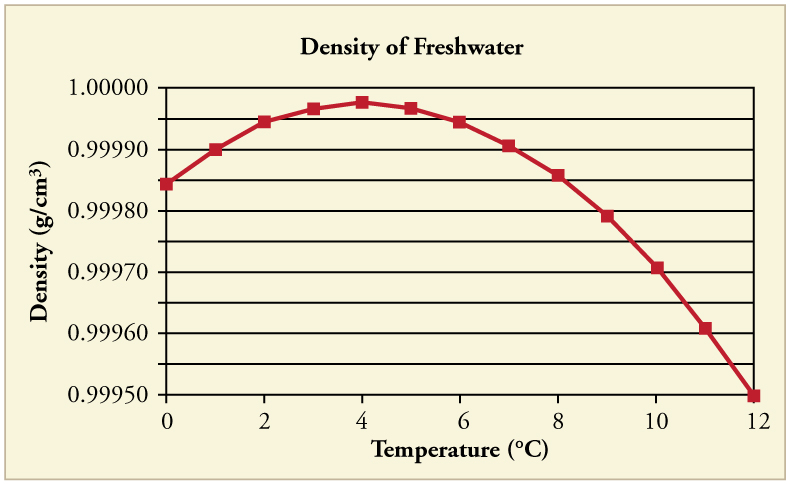Figure 3. The density of water as a function of temperature. Note that the thermal expansion is actually very small. The maximum density at +4ºC is only 0.0075% greater than the density at 2ºC, and 0.012% greater than that at 0ºC.

### Making Connections: Real-World Connections—Filling the Tank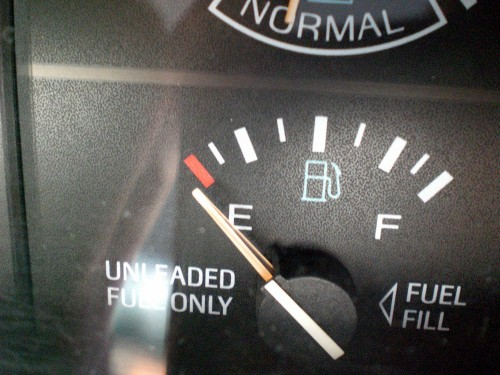Figure 4. Because the gas expands more than the gas tank with increasing temperature, you can’t drive as many miles on “empty” in the summer as you can in the winter. (credit: Hector Alejandro, Flickr)

Differences in the thermal expansion of materials can lead to interesting effects at the gas station. One example is the dripping of gasoline from a freshly filled tank on a hot day. Gasoline starts out at the temperature of the ground under the gas station, which is cooler than the air temperature above. The gasoline cools the steel tank when it is filled. Both gasoline and steel tank expand as they warm to air temperature, but gasoline expands much more than steel, and so it may overflow.

This difference in expansion can also cause problems when interpreting the gasoline gauge. The actual amount (mass) of gasoline left in the tank when the gauge hits “empty” is a lot less in the summer than in the winter. The gasoline has the same volume as it does in the winter when the “add fuel” light goes on, but because the gasoline has expanded, there is less mass. If you are used to getting another 40 miles on “empty” in the winter, beware—you will probably run out much more quickly in the summer.

### Example 2. Calculating Thermal Expansion: Gas vs. Gas Tank

Suppose your 60.0-L (15.9-gal) steel gasoline tank is full of gas, so both the tank and the gasoline have a temperature of 15.0ºC. How much gasoline has spilled by the time they warm to 35.0ºC?

#### Strategy

The tank and gasoline increase in volume, but the gasoline increases more, so the amount spilled is the difference in their volume changes. (The gasoline tank can be treated as solid steel.) We can use the equation for volume expansion to calculate the change in volume of the gasoline and of the tank.

#### Solution

1. Use the equation for volume expansion to calculate the increase in volume of the steel tank: ΔVs = βsVsΔT.
2. The increase in volume of the gasoline is given by this equation: ΔVgas = βgasVgasΔT.
3. Find the difference in volume to determine the amount spilled as VspillVgas − ΔVs.

Alternatively, we can combine these three equations into a single equation. (Note that the original volumes are equal.)

$\begin{array}{lll}{V}_{\text{spill}}& =& \left({\beta }_{\text{gas}}-{\beta }_{\text{s}}\right)V\Delta T\\ & =& \left[\left(\text{950}-\text{35}\right)\times {\text{10}}^{-6}/^{\circ}\text{C}\right]\left(\text{60}\text{.}0\text{L}\right)\left(\text{20}\text{.}0^{\circ}\text{C}\right)\\ & =& 1\text{.}\text{10}\text{L}\end{array}\\$

#### Discussion

This amount is significant, particularly for a 60.0-L tank. The effect is so striking because the gasoline and steel expand quickly. The rate of change in thermal properties is discussed in the chapter Heat and Heat Transfer Methods.

If you try to cap the tank tightly to prevent overflow, you will find that it leaks anyway, either around the cap or by bursting the tank. Tightly constricting the expanding gas is equivalent to compressing it, and both liquids and solids resist being compressed with extremely large forces. To avoid rupturing rigid containers, these containers have air gaps, which allow them to expand and contract without stressing them.

## Thermal Stress

Thermal stress is created by thermal expansion or contraction (see Elasticity: Stress and Strain for a discussion of stress and strain). Thermal stress can be destructive, such as when expanding gasoline ruptures a tank. It can also be useful, for example, when two parts are joined together by heating one in manufacturing, then slipping it over the other and allowing the combination to cool. Thermal stress can explain many phenomena, such as the weathering of rocks and pavement by the expansion of ice when it freezes.

### Example 3. Calculating Thermal Stress: Gas Pressure

What pressure would be created in the gasoline tank considered in Example 2, if the gasoline increases in temperature from 15.0ºC to 35.0ºC without being allowed to expand? Assume that the bulk modulus B for gasoline is 1.00 × 109 N/m2.

#### Strategy

To solve this problem, we must use the following equation, which relates a change in volume ΔV to pressure:

$\Delta{V}=\frac{1}{B}\frac{F}{A}V_0\\$

where $\frac{F}{A}\\$ is pressure, V0 is the original volume, and B is the bulk modulus of the material involved. We will use the amount spilled in Example 2 as the change in volume, ΔV.

#### Solution

1. Rearrange the equation for calculating pressure: $P=\frac{F}{A}=\frac{\Delta{V}}{V_0}B\\$.
2. Insert the known values. The bulk modulus for gasoline is B = 1.00 × 109 N/m2. In the previous example, the change in volume ΔV = 1.10 L is the amount that would spill. Here, V0 = 60.0 L is the original volume of the gasoline. Substituting these values into the equation, we obtain $P=\frac{1.10\text{ L}}{60.0\text{ L}}\left(1.00\times10^9\text{ Pa}\right)=1.83\times10^7\text{ Pa}\\$.

#### Discussion

This pressure is about 2500 lb/in2, much more than a gasoline tank can handle.

Forces and pressures created by thermal stress are typically as great as that in the example above. Railroad tracks and roadways can buckle on hot days if they lack sufficient expansion joints. (See Figure 5.) Power lines sag more in the summer than in the winter, and will snap in cold weather if there is insufficient slack. Cracks open and close in plaster walls as a house warms and cools. Glass cooking pans will crack if cooled rapidly or unevenly, because of differential contraction and the stresses it creates. (Pyrex® is less susceptible because of its small coefficient of thermal expansion.) Nuclear reactor pressure vessels are threatened by overly rapid cooling, and although none have failed, several have been cooled faster than considered desirable. Biological cells are ruptured when foods are frozen, detracting from their taste. Repeated thawing and freezing accentuate the damage. Even the oceans can be affected. A significant portion of the rise in sea level that is resulting from global warming is due to the thermal expansion of sea water.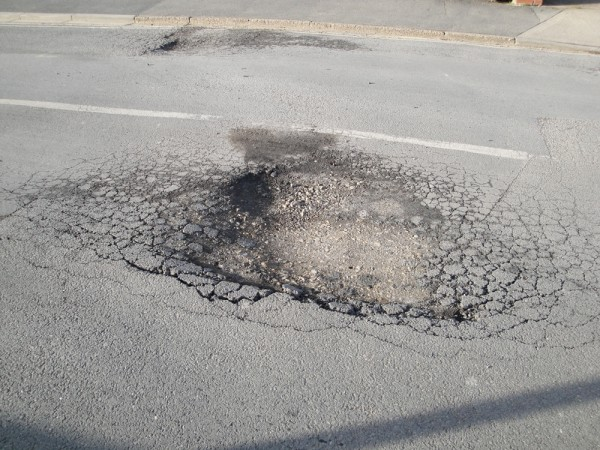Figure 5. Thermal stress contributes to the formation of potholes. (credit: Editor5807, Wikimedia Commons)

Metal is regularly used in the human body for hip and knee implants. Most implants need to be replaced over time because, among other things, metal does not bond with bone. Researchers are trying to find better metal coatings that would allow metal-to-bone bonding. One challenge is to find a coating that has an expansion coefficient similar to that of metal. If the expansion coefficients are too different, the thermal stresses during the manufacturing process lead to cracks at the coating-metal interface.

Another example of thermal stress is found in the mouth. Dental fillings can expand differently from tooth enamel. It can give pain when eating ice cream or having a hot drink. Cracks might occur in the filling. Metal fillings (gold, silver, etc.) are being replaced by composite fillings (porcelain), which have smaller coefficients of expansion, and are closer to those of teeth.

### Check Your Understanding

Two blocks, A and B, are made of the same material. Block A has dimensions × × × 2× L and Block B has dimensions 2× 2× 2L. If the temperature changes, what is

1. the change in the volume of the two blocks,
2. the change in the cross-sectional area l×w, and
3. the change in the height h of the two blocks?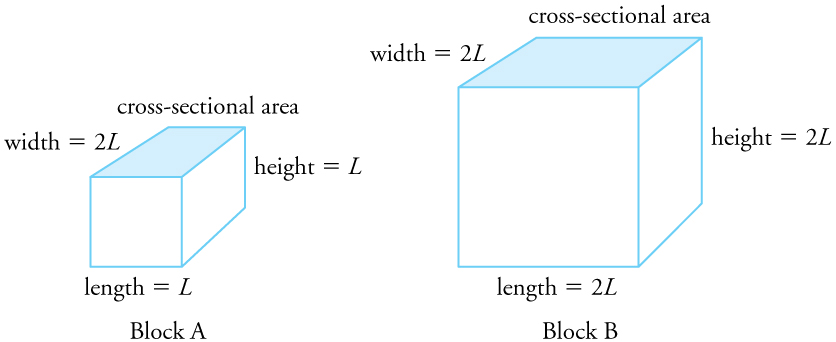Figure 6.

#### Solution

1. The change in volume is proportional to the original volume. Block A has a volume of × 2× = 2L3. Block B has a volume of 2× 2× 2= 8L3, which is 4 times that of Block A. Thus the change in volume of Block B should be 4 times the change in volume of Block A.
2. The change in area is proportional to the area. The cross-sectional area of Block A is × 2= 2L2, while that of Block B is 2× 2= 4L2. Because cross-sectional area of Block B is twice that of Block A, the change in the cross-sectional area of Block B is twice that of Block A.
3. The change in height is proportional to the original height. Because the original height of Block B is twice that of A, the change in the height of Block B is twice that of Block A.

## Section Summary

• Thermal expansion is the increase, or decrease, of the size (length, area, or volume) of a body due to a change in temperature.
• Thermal expansion is large for gases, and relatively small, but not negligible, for liquids and solids.
• Linear thermal expansion is Δ= αLΔT, where ΔL is the change in length L, ΔT is the change in temperature, and α is the coefficient of linear expansion, which varies slightly with temperature.
• The change in area due to thermal expansion is Δ= 2αAΔT, where ΔA is the change in area.
• The change in volume due to thermal expansion is Δ= βVΔT, where β is the coefficient of volume expansion and β ≈ 3α. Thermal stress is created when thermal expansion is constrained.

### Conceptual Questions

1. Thermal stresses caused by uneven cooling can easily break glass cookware. Explain why Pyrex®, a glass with a small coefficient of linear expansion, is less susceptible.
2. Water expands significantly when it freezes: a volume increase of about 9% occurs. As a result of this expansion and because of the formation and growth of crystals as water freezes, anywhere from 10% to 30% of biological cells are burst when animal or plant material is frozen. Discuss the implications of this cell damage for the prospect of preserving human bodies by freezing so that they can be thawed at some future date when it is hoped that all diseases are curable.
3. One method of getting a tight fit, say of a metal peg in a hole in a metal block, is to manufacture the peg slightly larger than the hole. The peg is then inserted when at a different temperature than the block. Should the block be hotter or colder than the peg during insertion? Explain your answer.
4. Does it really help to run hot water over a tight metal lid on a glass jar before trying to open it? Explain your answer.
5. Liquids and solids expand with increasing temperature, because the kinetic energy of a body’s atoms and molecules increases. Explain why some materials shrink with increasing temperature.

### Problems & Exercises

1. The height of the Washington Monument is measured to be 170 m on a day when the temperature is 35.0ºC. What will its height be on a day when the temperature falls to 10.0ºC? Although the monument is made of limestone, assume that its thermal coefficient of expansion is the same as marble’s.
2. How much taller does the Eiffel Tower become at the end of a day when the temperature has increased by 15ºC? Its original height is 321 m and you can assume it is made of steel.
3. What is the change in length of a 3.00-cm-long column of mercury if its temperature changes from 37.0ºC to 40.0ºC, assuming the mercury is unconstrained?
4. How large an expansion gap should be left between steel railroad rails if they may reach a maximum temperature 35.0ºC greater than when they were laid? Their original length is 10.0 m.
5. You are looking to purchase a small piece of land in Hong Kong. The price is “only” $60,000 per square meter! The land title says the dimensions are 20 m × 30 m. By how much would the total price change if you measured the parcel with a steel tape measure on a day when the temperature was 20ºC above normal? 6. Global warming will produce rising sea levels partly due to melting ice caps but also due to the expansion of water as average ocean temperatures rise. To get some idea of the size of this effect, calculate the change in length of a column of water 1.00 km high for a temperature increase of 1.00ºC. Note that this calculation is only approximate because ocean warming is not uniform with depth. 7. Show that 60.0 L of gasoline originally at 15.0ºC will expand to 61.1 L when it warms to 35.0ºC, as claimed in Example 2. 8. (a) Suppose a meter stick made of steel and one made of invar (an alloy of iron and nickel) are the same length at 0ºC. What is their difference in length at 22.0ºC? (b) Repeat the calculation for two 30.0-m-long surveyor’s tapes. 9. (a) If a 500-mL glass beaker is filled to the brim with ethyl alcohol at a temperature of 5.00ºC, how much will overflow when its temperature reaches 22.0ºC? (b) How much less water would overflow under the same conditions? 10. Most automobiles have a coolant reservoir to catch radiator fluid that may overflow when the engine is hot. A radiator is made of copper and is filled to its 16.0-L capacity when at 10.0ºC. What volume of radiator fluid will overflow when the radiator and fluid reach their 95.0ºC operating temperature, given that the fluid’s volume coefficient of expansion is β = 400 × 10–6/ºC? Note that this coefficient is approximate, because most car radiators have operating temperatures of greater than 95.0ºC. 11. A physicist makes a cup of instant coffee and notices that, as the coffee cools, its level drops 3.00 mm in the glass cup. Show that this decrease cannot be due to thermal contraction by calculating the decrease in level if the 350cm3 of coffee is in a 7.00-cm-diameter cup and decreases in temperature from 95.0ºC to 45.0ºC. (Most of the drop in level is actually due to escaping bubbles of air.) 12. (a) The density of water at 0ºC is very nearly 1000kg/m3 (it is actually 999.84 kg/m3), whereas the density of ice at 0ºC is 917 kg/m3. Calculate the pressure necessary to keep ice from expanding when it freezes, neglecting the effect such a large pressure would have on the freezing temperature. (This problem gives you only an indication of how large the forces associated with freezing water might be.) (b) What are the implications of this result for biological cells that are frozen? 13. Show that β ≈ 3α, by calculating the change in volume ΔV of a cube with sides of length L. ## Glossary thermal expansion: the change in size or volume of an object with change in temperature coefficient of linear expansion: α, the change in length, per unit length, per 1ºC change in temperature; a constant used in the calculation of linear expansion; the coefficient of linear expansion depends on the material and to some degree on the temperature of the material coefficient of volume expansion: β, the change in volume, per unit volume, per 1ºC change in temperature thermal stress: stress caused by thermal expansion or contraction ### Selected Answers to Problems & Exercises 1. 169.98 m 3. 5.4 × 10−6 m 5. Because the area gets smaller, the price of the land DECREASES by ~$17,000.

7. $\begin{array}{lll}V& =& {V}_{0}+\Delta V={V}_{0}\left(1+\beta \Delta T\right)\\ & =& \left(\text{60}\text{.}\text{00 L}\right)\left[1+\left(\text{950}\times {\text{10}}^{-6}/^{\circ}\text{C}\right)\left(\text{35}\text{.}0^{\circ}\text{C}-\text{15}\text{.}0^{\circ}\text{C}\right)\right]\\ & =& \text{61}\text{.}1\text{L}\end{array}\\$

9. (a) 9.35 mL; (b) 7.56 mL

11. 0.832 mm

13. We know how the length changes with temperature: Δ= αL0ΔT. Also we know that the volume of a cube is related to its length by = L3, so the final volume is then = V+ Δ= (L0 + ΔL)3. Substituting for ΔL gives = (L0 + αL0ΔT)= L03(1 + αΔT)3.

Now, because αΔT is small, we can use the binomial expansion: L03(1 + 3αΔT) = L0+ 3αL03ΔT.

So writing the length terms in terms of volumes gives = V+ ΔV+ 3αV0ΔT, and so Δ=βV0Δ≈ 3αV0ΔT, or β ≈ 3α.

1. Values for liquids and gases are approximate.